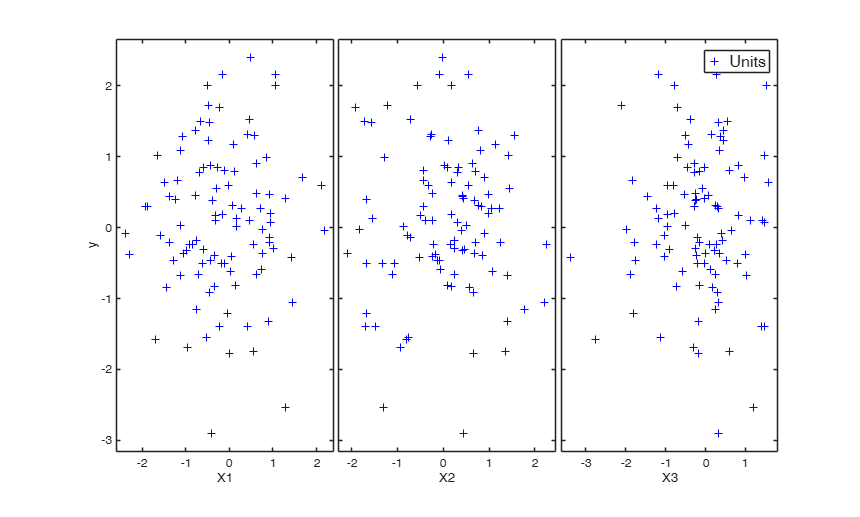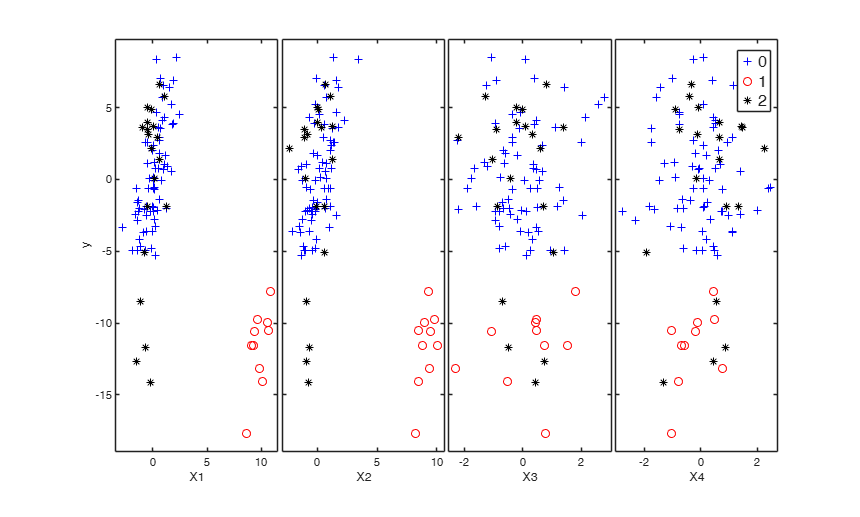# simulateLM

simulateLM simulates linear regression data with prespecified values of statistical indexes.

## Syntax

• out=simulateLM(n)example
• out=simulateLM(n,Name,Value)example

## Description

simulateLM simulates linear regression data. It is possible to specify: 1) the requested value of R2 (or equivaletly its SNR);

2) the values of the beta coefficients (possibly sparse);

3) the correlation (covariance) matrix among the explanatory variables.

4) the value of the intercept term.

5) the distribution to use to generate the Xs;

6) the distribution to use to generate the ys.

7) the MSOM contamination in Xs and ys.

8) the VIOM contamination in ys.

 out =simulateLM(n) Use all defaul options.

 out =simulateLM(n, Name, Value) Simulate with prefixed value of R2.

## Examples

expand all

###Use all defaul options.

Simulate 100 observations y and X (uncorrelated with y) using standard normal distribution.

out=simulateLM(100,'plots',true);###Simulate with prefixed value of R2.

Set value of R2;

R2=0.82;
n=10000;
out=simulateLM(n,'R2',R2);
outLM=fitlm(out.X,out.y);

## Related Examples

expand all

###Use prefixed correlation matrix for cov(X).

Set value of R2;

R2=0.26;
n=10000;
A = gallery('moler',5,0.2);
out=simulateLM(n,'R2',R2,'SigmaX',A);
outLM=fitlm(out.X,out.y)
outLM =

Linear regression model:
y ~ 1 + x1 + x2 + x3 + x4 + x5

Estimated Coefficients:
Estimate        SE        tStat       pValue
_________    ________    _______    __________

(Intercept)    -0.076653    0.053898    -1.4222       0.15501
x1                1.0515    0.056414     18.638    3.0647e-76
x2               0.92447    0.056001     16.508    2.0145e-60
x3                1.0394    0.055297     18.797      1.72e-77
x4               0.92012    0.055248     16.654    1.8903e-61
x5                 1.029    0.054653     18.828    9.8022e-78

Number of observations: 10000, Error degrees of freedom: 9994
Root Mean Squared Error: 5.39
F-statistic vs. constant model: 679, p-value = 0


###Use prefixed values of R2, beta and intercept.

Set value of R2.

R2=0.92;
beta=[3; 4; 5; 2; 7];
intercept=true;
n=100000;
out=simulateLM(n,'R2',R2,'beta',beta);
outLM=fitlm(out.X,out.y);

### Sim study.

Compare the distribution of values of R2 with data generated from Normal with those generated from Student T with 5 degrees of freedom.

% Set value of R2.
R2=0.92;
beta=[3; 4; 5; 2; 7; 2; 3];
nsimul=1000;
R2all=zeros(nsimul,2);
n=100;
df=5;
for j=1:nsimul
% Data generated from Normal
out=simulateLM(n,'R2',R2,'beta',beta);
outLM=fitlm(out.X,out.y);
R2all(j,1)=outLM.Rsquared.Ordinary;
% Data generated from T(5)
out=simulateLM(n,'R2',R2,'beta',beta,'distriby','T','distribypars',df);
outLM=fitlm(out.X,out.y);
R2all(j,2)=outLM.Rsquared.Ordinary;
end
boxplot(R2all,'Labels',{'Normal', 'T(5)'});

###Use SNR and include MSOM (on active features) and VIOM contamination SNR=3; beta=[2, 2, 0, 0]; intercept=true; n=100; out=simulateLM(n,'SNR',SNR,'beta',beta, 'pMSOM', 0.

%% Use SNR and include MSOM (on active features) and VIOM contamination
SNR=3;
beta=[2, 2, 0, 0];
intercept=true;
n=100;
out=simulateLM(n,'SNR',SNR,'beta',beta, 'pMSOM', 0.1, 'pVIOM', 0.2, 'plots', 1);
X = out.X;
y = out.y;
outLM=fitlm(X,y);
Xc = out.Xc;
yc = out.yc;
outLM2=fitlm(Xc,yc);## Input Arguments

### n — sample size. Scalar.

n is a positive integer which defines the length of the simulated data. For example if n=100, y will be 100x1 and X will be 100xp.

Data Types: single| double

### Name-Value Pair Arguments

Specify optional comma-separated pairs of Name,Value arguments. Name is the argument name and Value is the corresponding value. Name must appear inside single quotes (' '). You can specify several name and value pair arguments in any order as  Name1,Value1,...,NameN,ValueN.

Example:  'R2',0.90 , 'SNR',10 , 'beta',[3 5 8] , 'Sigma', gallery('lehmer',5) , 'distribX', 'Beta' , 'distribXpars', '[0.2 0.6]' , 'distriby', 'Lognormal' , 'distribypars', '[2 10]' , 'distribypars', '[2 10]' , 'intercept', true , 'plots',false , 'pMSOM',0.25 , 'pVIOM',0.25 , 'shiftMSOMe',-3 , 'predMSOM',true(2,1) , 'shiftMSOMx',3 , 'inflVIOMe',5 

### R2 —Squared multiple correlation coefficient (R2).scalar.

The requested value of R2. A number in the interval [0 1] which specifies the requested value of R2.

The default is to simulate regression data with R2=0;

Example:  'R2',0.90 

Data Types: double

### SNR —Signal to noise ratio characterizing the simulation.this is defined such that sigma_error == sqrt(var(X_u*beta_true)/SNR) The default is SNR=='' and R2 is used instead.

Example:  'SNR',10 

Data Types: double

### beta —the values of the beta coefficients.vector.

Vector which contains the values of the regression coefficients. The default is a vector of ones.

Example:  'beta',[3 5 8] 

Data Types: double

### SigmaX —the correlation matrix.matrix.

Positive definite matrix which contains the correlation matrix among regressors. The default is the identity matrix.

Example:  'Sigma', gallery('lehmer',5) 

Data Types: double

### distribX —distribution to use to simulate the regressors.character.

Character which specifies the distribution to use to simulate the values of the explanatory variables.

For the list of valid names see MATLAB function random.

Default is to use the Standard normal distribution.

Example:  'distribX', 'Beta' 

Data Types: double

### distribXpars —parameters of the distribution to use in distribX.vector.

Scalar value or array of scalar values containing the distribution parameters specified in distribX.

Example:  'distribXpars', '[0.2 0.6]' 

Data Types: double

### distriby —distribution to use to simulate the response.character.

Character which specifies the distribution to use to simulate the values of the explanatory variables. The default is to use the Standard normal distribution.

Example:  'distriby', 'Lognormal' 

Data Types: double

### distribypars —parameters of the distribution to use in distriby.vector.

Scalar value or array of scalar values containing the distribution parameters specified in distriby. For examples if distriby is 'Lognormal' and 'distribypars' is [2 10], the errors are generated according to a Log Normal distribution with parameters mu and sigma respectively equal to 2 and 10.

Example:  'distribypars', '[2 10]' 

Data Types: double

### nexpl —number of explanatory variables.if vector beta is supplied nexpl is equal to length(beta).

Similarly if sigmaX is supplied nexpl is set equal to size(sigmaX,1).

Note that both nexpl is supplied together with beta and SigmaX it is check that nexpl =length(beta) = size(SigmaX,1). If options beta and sigmaX are empty nexpl is set equal to 3.

Example:  'distribypars', '[2 10]' 

Data Types: double

### intercept —value of the intercept to use.boolean.

The default value for intercept is false.

Example:  'intercept', true 

Data Types: boolean

### plots —Plot on the screen.boolean.

If plots = true, the yXplot which shows the response against all the explanatory variables s shown on the screen. The default value for plots is false, that is no plot is shown on the screen.

Example:  'plots',false 

Data Types: single | double

### pMSOM —Proportion of MSOM outliers.the default is 10% MSOM contmaination.

Example:  'pMSOM',0.25 

Data Types: double

### pVIOM —Proportion of VIOM outliers (non-overlapping with MSOM).the default is 10% VIOM contmaination.

Example:  'pVIOM',0.25 

Data Types: double

### shiftMSOMe —Mean-shift on the error terms for MSOM outliers.default value shiftMSOMe==10.

Example:  'shiftMSOMe',-3 

Data Types: double

### predxMSOM —Predictors subject to a mean shift by MSOM.it is a p-dimensional vector indexing design matrix columns.

Default value is to contaminate only the non-zero entries of beta_true (excluding the intercept).

Example:  'predMSOM',true(2,1) 

Data Types: boolean

### shiftMSOMx —Mean-shift on the predictor terms for MSOM outliers.default value shiftMSOMx==10.

Example:  'shiftMSOMx',3 

Data Types: double

### inflVIOMe —Variance-inflation for the errors subject to a VIOM.default value is inflVIOMe==10.

Example:  'inflVIOMe',5 

Data Types: double

## Output Arguments

### out — description Structure

Structure which contains the following fields

Value Description
y

simulated response. Vector. Column vector of length n containing the response.

X

simulated regressors. Matrix . Matrix of size n-times-nexpl containing the values of the regressors.

Optional Output (for pVIOM+pMSOM>0):

yc

Contaminated response vector.

Xc

Contaminated response vector.

ind_clean

Indexes for non-outlying cases.

ind_MSOM

Indexes for MSOM outlying cases.

ind_VIOM

Indexes for VIOM outlying cases.

vareps

Variance for the uncontaminated errors.

Insolia, L., F. Chiaromonte, and M. Riani (2020a).

"A Robust Estimation Approach for Mean-Shift and Variance-Inflation Outliers".

Festschrift in Honor of R. Dennis Cook pp 17–41.